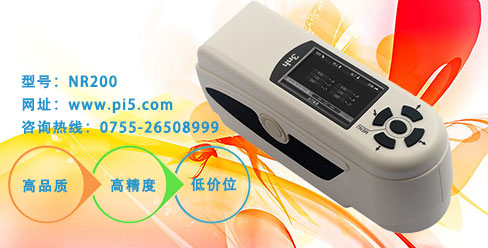# 畅销的色彩色差仪是如何计算色差的

畅销的色彩色差仪是如何计算色差的ANLAB色差公式

△E=40{[△(Vx-Vy)]2+(0.23△Vy)+[0.4△(Vz+Vy)]2}1/2

CIE L*a*b* (CIE LAB)色差公式

CIE LAB计算公式为：

L*=116(Y/Y0)1/3-16

a*=500{(X/X0)1/3-(Y/Y0)1/3}

b*=200{(Y/Y0)1/3-(Y/Y0)1/3}

X0=94.825；Y0=100.000；Z0=107.381

X0、Y0、Z0为理想白色物体的D65光源100°视场色彩色差仪的三刺激值。

C=(a2+b2)1/2

CMC(1:C)色差

CMC(1:C) 色差公式 ：

△ECMC={(△L*／I•SL)2 + (△C*／C•SC) 2+(△H*／SH)2}1/2

CMC

JPC79色差式

△E=[(△L/Lt)2+(△C/Ct)2+(△C/Ct)]2+(△H/Ht)2]1/2

Ct = 0.06380C/(1+ 0.01310C)+0.638

Ht = tnCt；tn=tf+1-f

△L——由ANLAB色差式计算得到的亮度值；

△H——由ANLAB色差式计算得到的色相差；

△C——由ANLA B色差式计算得到的饱和度差。

FMCⅡ式

△E=[(△C)2+ (△L)2]1/2

K1 = 0.5569+0.049434Y-0.82575X10-3Y2+0.79172X10-5Y3-0.30087X10-7Y4

K2= 0.17548+0.027556Y- 0.57262×10-3Y2+0.63893×10-5Y3-0.26730×1 0-7Y4

Hunter公式

L=100(Y/Y0)1/2

a=Ka(X/X0-Y/Y0)/(Y/Y0)1/2

b=Kb(Y/Y0-Z/Z0)/( Y/Y0)1/2

Ka、Kb——照明体系数 。

## 浏览历史

Pi5红包

### 关注微博 优惠活动不容错过！微信二维码扫描